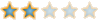Montreal Math ClubMathematics students in Montreal - Étudiants de mathématiques à MontréalHomeCalendarFAQSearchMemberlistUsergroupsRegisterLog in

 Montreal Math Club :: Mathematics :: Geometry Share

four-dimensional synthetic geometryAuthorMessage

EuclidPosts : 95
Join date : 2009-09-15
Age : 57
Location : AlexandriaSubject: four-dimensional synthetic geometryMon Sep 28, 2009 8:44 am There is a hyper-polyhedron bounded by six regular right triangular prisms in the 4-dimensions space. All nine edges of a prism equal to 1. Calculate the radius of the circumscribed hyper-sphere.four-dimensional synthetic geometryPage 1 of 1

Permissions in this forum:You cannot reply to topics in this forumJump to: Select a forum||--Math Club|   |--Mathematical Sundays|   |--Suggestions|   |--Introduce yourself|   |--Mathematics|   |--Problem Solving|   |   |--Solved problems|   |   |   |--Calculus and Analysis|   |--Combinatorics|   |--Geometry|   |--Number Theory|   |--Probabilities and statistics|   |--Set theory and Topology|   |--Online Resources|   |--Chit chat    |--The Water Cooler    |--Mathematical poems